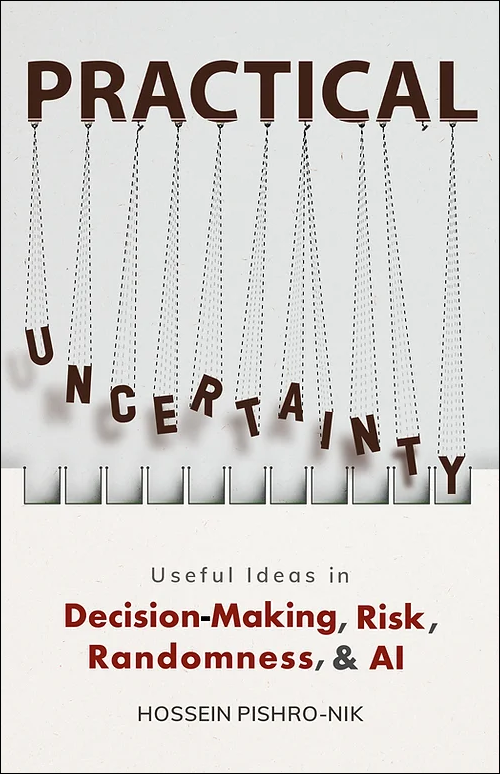## 1.1.0 Introduction: What Is Probability?

Randomness and uncertainty exist in our daily lives as well as in every discipline in science, engineering, and technology. Probability theory, the subject of the first part of this book, is a mathematical framework that allows us to describe and analyze random phenomena in the world around us. By random phenomena, we mean events or experiments whose outcomes we can't predict with certainty.

Let's consider a couple of specific applications of probability in order to get some intuition. First, let's think more carefully about what we mean by the terms "randomness" and "probability" in the context of one of the simplest possible random experiments: flipping a fair coin.

One way of thinking about "randomness" is that it's a way of expressing what we don't know. Perhaps if we knew more about the force I flipped the coin with, the initial orientation of the coin, the impact point between my finger and the coin, the turbulence in the air, the surface smoothness of the table the coin lands on, the material characteristics of the coin and the table, and so on, we would be able to definitively say whether the coin would come up heads or tails. However, in the absence of all that information, we cannot predict the outcome of the coin flip. When we say that something is random, we are saying that our knowledge about the outcome is limited, so we can't be certain what will happen.

Since the coin is fair, if we don't know anything about how it was flipped, the probability that it will come up heads is 50%, or $\frac{1}{2}$. What exactly do we mean by this? There are two common interpretations of the word "probability." One is in terms of relative frequency. In other words, if we flip the coin a very large number of times, it will come up heads about $\frac{1}{2}$ of the time. As the number of coin flips increases, the proportion that come up heads will tend to get closer and closer to $\frac{1}{2}$. In fact, this intuitive understanding of probability is a special case of the law of large numbers, which we will state and prove formally in later chapters of the book.

A second interpretation of probability is that it is a quantification of our degree of subjective personal belief that something will happen. To get a sense of what we mean by this, it may be helpful to consider a second example: predicting the weather. When we think about the chances that it will rain today, we consider things like whether there are clouds in the sky and the humidity. However, the beliefs that we form based on these factors may vary from person to person - different people may make different estimates of the probability that it will rain. Often these two interpretations of probability coincide - for instance, we may base our personal beliefs about the chance that it will rain on an assessment of the relative frequency of rain on days with conditions like today.

The beauty of probability theory is that it is applicable regardless of the interpretation of probability that we use (i.e., in terms of long-run frequency or degree of belief). Probability theory provides a solid framework to study random phenomena. It starts by assuming axioms of probability, and then building the entire theory using mathematical arguments.

Before delving into studying probability theory, let us briefly look at an example showing how probability theory has been applied in a real life system.

 The print version of the book is available on Amazon.Practical uncertainty: Userful Ideas in Decision-Making, Risk, Randomness, & AI